We think you are located in United States. Is this correct?

# 5.2 Work

## 5.2 Work (ESCMB)

We cover different topics in different chapters in different grades but that doesn't mean that they are not related. In fact, it is very important to note that all of the different topics related to mechanics (forces, mechanical energy, momentum, rectilinear motion) actually form a consistent picture of the same physical system. There have been examples where we've shown the same results using two methods, for example determining speed or velocity using equations of motion or conservation of mechanical energy. Learning about work will help us tie everything we've learnt about previously together.

Work will allow us to connect energy transfer to forces, which we have already linked to momentum and the equations of motion. When a force tends to act in or against the direction of motion of an object we say that the force is doing work on the object. Specifically, work is defined mathematically in terms of the force and the displacement of the object.

Work

When a force acts in or against the direction of motion of an object, work is done on the object.

$W=F\Delta x\cos\theta$

This means that in order for work to be done, an object must have its position changed by an amount $$\Delta \vec{x}$$ while a force, $$\vec{F}$$, is acting on it such that there is some non-zero component of the force in the direction of the displacement. Work is calculated as:

$W=F\Delta x\cos\theta$

Where:

• $$W$$ = work in joules (J)
• $$F$$ = magnitude of force for which we are calculating work in newtons (N)
• $$\Delta x$$ = magnitude of displacement in metres (m)
• $$\theta$$ = angle between the force and the direction of displacement

Note that:

• $$F\cos\theta$$ is the magnitude of the component of $$\vec{F}$$ in the direcion of $$\Delta \vec{x}$$. If $$\theta > 90^{o}$$ then the component is parallel to the direction of displacement but point in the opposite direction and the force is opposing the motion.
• We will deal with constant forces not forces that are continuously changing in this chapter. All of the principles we have covered are applicable when forces are not constant.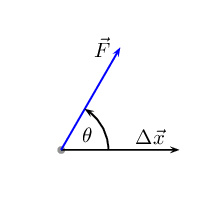Figure 5.1: The force $$\vec{F}$$ causes the object to be displaced by $$\Delta \vec{x}$$ at angle θ.

Important: $$\cos\theta$$ tells you the relative direciton of the force and the displacment which is important. If the component of the force along the direction of the displacement is opposite in direction to the displacement then the sign of the displacement vector and force vector will be different. This is regardless of which direction was chosen as a positive direction.

Let us look at some examples to understand this properly. In the images below the grey dot represents an object. A force, $$\vec{F}$$, acts on the object. The object moves through a displacement, $$\Delta \vec{x}$$. What is the sign of the work done in each case?

At $$\theta=90^\circ$$ the magnitude of the component of the force in the direction of the displacement is zero so no work is done as $$\cos(90)=0$$.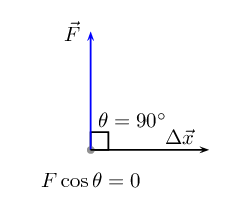At $$\theta>90^\circ$$ the magnitude of the component of the force along the line of the displacement is opposite in direction to the displacement so negative work is done, $$\cos\theta < 0$$.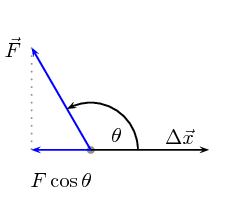It is very important to note that for work to be done there must be a component of the applied force in the direction of motion. Forces perpendicular to the direction of motion do no work.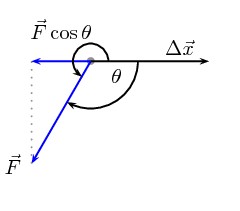Figure 5.2: The force $$\vec{F}$$ causes the object to be displaced by $$\Delta \vec{x}$$ at angle θ.

It is only the direction of the force on the object that matters and not the direction from the source of the force to the object. In Figure 5.3 both powerlifters are exerting an upwards force on the weights. On the left the weight is being pulled upwards and on the right it is being pushed upwards.

Weight lifting is a good context in which to think about work because it helps to identify misconceptions introduced by everyday use of the term `work'. In the two cases in Figure 5.3 everyone would describe moving the weights upwards as very hard work. From a physics perspective, as the powerlifters lift the weight they are exerting a force in the direction of the displacement so positive work is done on the weights.

Consider the strongman walking in Figure 5.4. He carries two very heavy sleds as far as he can in a competition. What work is the man doing on the sleds and why?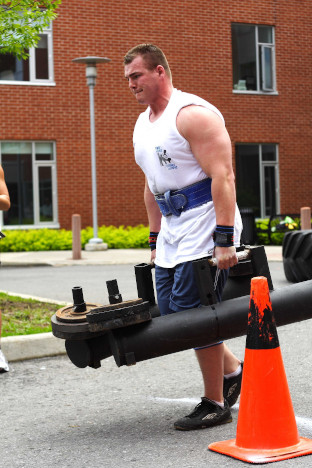Figure 5.4: A strongman carries heavy sleds as far as possible in a competition.

Most people would say he is working very hard because the sleds are heavy to carry but from a physics perspective he is doing no work on the sleds. The reason that he does no work is because the force he exerts is directly upwards to balance the force of gravity and the displacement is in the horizontal direction. Therefore there is no component of the force in the direction of displacement ($$\theta=90^\circ$$) and no work done.

His muscles do need to use their energy reserves to maintain the force to balance gravity. That does not result in energy transfer to the sleds.

## Is work done?

Decide whether or not work is done in the following situations. Remember that for work to be done a force must be applied in the direction of motion and there must be a displacement. Identify which two objects are interacting, what the action-reaction pairs of forces are and why the force described is or isn't doing work.

1. Max pushes against a wall and becomes tired.

2. A book falls off a table and free falls to the ground.

3. A rocket accelerates through space.

4. A waiter holds a tray full of plates above his head with one arm and carries it straight across the room at constant speed.

1. Max pushes against a wall and becomes tired. Answer: No work is done because there is no displacement.

2. A book falls off a table and free falls to the ground. Answer: Yes work is done because there is a displacement in the direction of the force of gravity.

3. A rocket accelerates through space. Answer: Yes work is done because there is a net force acting for there to be a net acceleration. If there is an acceleration then there is a displacement.

4. A waiter holds a tray full of meals above his head with one arm and carries it straight across the room at constant speed. (Careful! This is a tricky question.) Answer: No work is done because there is no net force in the direction of the displacement.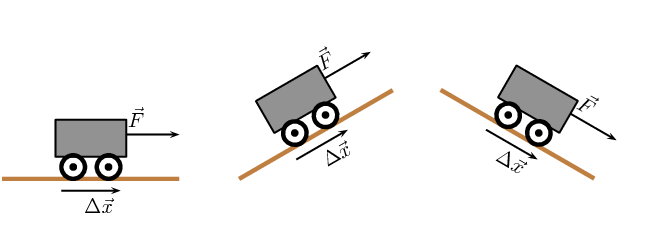For each of the above pictures, the force vector is acting in the same direction as the displacement vector. As a result, the angle $$\theta =0°$$ because there is no difference in angle between the direction of applied force and the direction of displacement.

The work done by a force can then be positive or negative. This sign tells us about the direction of the energy transfer. Work is a scalar so the sign should not be misinterpreted to mean that work is a vector. Work is defined as energy transfer, energy is a scalar quantity and the sign indicates whether energy was increased or decreased.

• If$$\vec{F}_{\text{applied}}$$ acts or has a component acting in the same direction as the motion, then positive work is being done. In this case the object on which the force is applied gains energy.

• If the direction of motion and $$\vec{F}_{\text{applied}}$$ are opposite, then negative work is being done. This means that energy is lost and the object exerting the force gains energy. For example, if you try to push a car uphill by applying a force up the slope and instead the car rolls down the hill you are doing negative work on the car. Alternatively, the car is doing positive work on you!

The everyday use of the word "work" differs from the physics use. In physics, only the component of the applied force that is parallel to the motion does work on an object. So, for example, a person holding up a heavy book does no work on the book.

As with all physical quantities, work must have units. Following from the definition, work is measured in $$\text{N·m}$$. The name given to this combination of S.I. units is the joule (symbol J).

## Worked example 1: Calculating work on a car when speeding up.

A car is travelling along a straight horizontal road. A force of $$\text{500}$$ $$\text{N}$$ is applied to the car in the direction that it is travelling, speeding it up. While it is speeding up is covers a distance of $$\text{20}$$ $$\text{m}$$. Calculate the work done on the car.### Analyse the question to determine what information is provided

• The magnitude of the force applied is $$F=\text{500}\text{ N}$$.

• The distance moved is $$\Delta x$$ = $$\text{20}$$ $$\text{m}$$.

• The applied force and distance moved are in the same direction. Therefore, the angle between the force and displacement is $$\theta=0^\circ$$.

These quantities are all in SI units, so no unit conversions are required.

### Analyse the question to determine what is being asked

• We are asked to find the work done on the car. We know from the definition that work done is $$W={F}\Delta x\cos\theta$$.

### Next we substitute the values and calculate the work done

\begin{align*} W& = {F}\Delta x\cos\theta\\ & = \left(500\right)\left(20\right) \left(\cos 0\right)\\ & = \left(500\right)\left(20\right) \left(1\right)\\ & = \text{10 000}\text{ J} \end{align*}

Remember that the answer must be positive, as the applied force and the displacement are in the same direction. In this case, the car gains kinetic energy.

## Worked example 2: Calculating work on the car while braking

The same car now slows down when a force of $$\text{300}$$ $$\text{N}$$ is applied opposite to the direction of motion while it travels $$\text{25}$$ $$\text{m}$$ forward. Calculate the work done on the car.### Analyse the question to determine what information is provided

• The magnitude of the force applied is $$F=\text{300}\text{ N}$$.

• The distance moved is $$\Delta x$$ = $$\text{25}$$ $$\text{m}$$.

• The applied force and distance moved are in the opposite direction. Therefore, the angle between the force and displacement is $$\theta=180^\circ$$.

These quantities are all in the correct units, so no unit conversions are required.

### Analyse the question to determine what is being asked

• We are asked to find the work done on the car. We know from the definition that work done is $$W={F}\Delta x\cos\theta$$

### Next we substitute the values and calculate the work done

\begin{align*} W& = {F}\Delta x\cos\theta\\ & = \left(300\right)\left(25\right) \left(\cos 180\right)\\ & = \left(300\right)\left(25\right) \left(-1\right)\\ & = -\text{7 500}\text{ J} \end{align*}

Note that the answer must be negative as the applied force and the displacement are in opposite directions. This means that the energy is being lost by the car. This may be energy lost as heat to the environment. Energy conservation still holds, the energy has just been transferred to a larger system that contains the car.

What happens when the applied force and the motion are not parallel? By using the formula $$W=F\Delta x\cos\theta$$ , we are actually calculating the component of the applied force in the direction of motion. Note that the component of the force perpendicular to the direction of motion does no work.

## Worked example 3: Calculating work done on a box pulled at an angle.

Calculate the work done on a box, if it is pulled $$\text{5}$$ $$\text{m}$$ along the ground by applying a force of $$F=\text{20}\text{ N}$$ at an angle of $$\text{60}$$$$\text{°}$$ to the horizontal.

### Analyse the question to determine what information is provided

• The force applied is $$F$$=$$\text{20}$$ $$\text{N}$$

• The distance moved is $$\Delta x$$ = $$\text{5}$$ $$\text{m}$$ along the ground

• The angle between the applied force and the motion is $$\theta$$=$$\text{60}$$$$\text{°}$$

These quantities are in the correct units so we do not need to perform any unit conversions.

### Analyse the question to determine what is being asked

• We are asked to find the work done on the box.

### Substitute and calculate the work done

Now we can calculate the work done on the box:

\begin{align*} W& = {F}\Delta x\cos\theta\\ & = \left(20 \right)\left(5 \right) \left(\cos 60\right)\\ & = \text{50}\text{ J} \end{align*}

Note that the answer is positive as the component of the force parallel to the direction of motion is in the same direction as the motion.

The work done on the box is $$\text{50}$$ $$\text{J}$$.

## Work

Textbook Exercise 5.1

A $$\text{10}$$ $$\text{N}$$ force is applied to push a block across a frictionless surface for a displacement of $$\text{5,0}$$ $$\text{m}$$ to the right. The block has a weight $$\vec{F}_{g }$$ of $$\text{20}$$ $$\text{N}$$. Determine the work done by the following forces: normal force, weight $$\vec{F}_{g }$$, applied force.Normal force acts perpendicular and therefore does no work.

The weight acts perpendicular to the direction of motion and does no work.

The applied force: \begin{align*} W_{\text{applied}}&= F\Delta x \cos\theta \\ &= (10)(5) \\ & = 50~\text{J} \end{align*}

Normal force:0 J, weight: 0 J, applied force: 50 J.

A $$\text{10}$$ $$\text{N}$$ frictional force slows a moving block to a stop after a displacement of $$\text{5,0}$$ $$\text{m}$$ to the right. The block has a weight of $$\text{20}$$ $$\text{N}$$ Determine the work done by the following forces: normal force, weight, frictional force.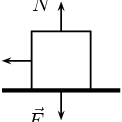Normal force acts perpendicular and therefore does no work.

The weight acts perpendicular to the direction of motion and does no work.

The frictional force: \begin{align*} W_{friciton}&= F\Delta x \cos\theta \\ &= (10)(5)\cos(180) \\ & = -50~\text{J} \end{align*}

Normal force:0 J, weight: 0 J, frictional force: -50 J.

A $$\text{10}$$ $$\text{N}$$ force is applied to push a block across a frictional surface at constant speed for a displacement of $$\text{5,0}$$ $$\text{m}$$ to the right. The block has a weight of $$\text{20}$$ $$\text{N}$$ and the frictional force is $$\text{10}$$ $$\text{N}$$. Determine the work done by the following forces: normal force, weight, applied force and frictional force.Normal force acts perpendicular and therefore does no work.

The weight acts perpendicular to the direction of motion and does no work.

The frictional force: \begin{align*} W_{friciton}&= F\Delta x \cos\theta \\ &= (10)(5)\cos(180) \\ & = -50~\text{J} \end{align*}

The applied force: \begin{align*} W_{\text{applied}}&= F\Delta x \cos\theta \\ &= (10)(5) \\ & = 50~\text{J} \end{align*}

Normal force:0 J, weight: 0 J, frictional force: -50 J, applied force: 50 J.

An object with a weight of $$\text{20}$$ $$\text{N}$$ is sliding at constant speed across a frictionless surface for a displacement of $$\text{5}$$ $$\text{m}$$ to the right. Determine if there is any work done.No work is done as all the forces act perpendicular to the direction of displacement.

An object with a weight of $$\text{20}$$ $$\text{N}$$ is pulled upward at constant speed by a $$\text{20}$$ $$\text{N}$$ force for a vertical displacement of $$\text{5}$$ $$\text{m}$$. Determine if there is any work done.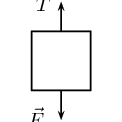The applied force: \begin{align*} W_{\text{applied}}&= F\Delta x \cos\theta \\ &= (20)(5) \\ & = 100~\text{J} \end{align*}

The gravitational force: \begin{align*} W_{gravitational}&= F\Delta x \cos\theta \\ &= (20)(5)\cos(180) \\ &= -(20)(5) \\ & = -100~\text{J} \end{align*}

Work done by applied force is 100 J, work done by gravity is -100 J

Before beginning its descent, a roller coaster is always pulled up the first hill to a high initial height. Work is done on the roller coaster to achieve this initial height. A coaster designer is considering three different incline angles of the hill at which to drag the $$\text{2 000}$$ $$\text{kg}$$ car train to the top of the $$\text{60}$$ $$\text{m}$$ high hill. In each case, the force applied to the car will be applied parallel to the hill. Her critical question is: which angle would require the least work? Analyse the data, determine the work done in each case, and answer this critical question.

 Angle of Incline Applied Force Distance Work $$\text{35}$$$$\text{°}$$ $$\text{1,1} \times \text{10}^{\text{4}}$$ $$\text{N}$$ $$\text{100}$$ $$\text{m}$$ $$\text{45}$$$$\text{°}$$ $$\text{1,3} \times \text{10}^{\text{4}}$$ $$\text{N}$$ $$\text{90}$$ $$\text{m}$$ $$\text{55}$$$$\text{°}$$ $$\text{1,5} \times \text{10}^{\text{4}}$$ $$\text{N}$$ $$\text{80}$$ $$\text{m}$$
 Angle of Incline Applied Force Distance Work $$\text{35}$$$$\text{°}$$ $$\text{1,1} \times \text{10}^{\text{4}}$$ $$\text{N}$$ $$\text{100}$$ $$\text{m}$$ $$\text{9,01} \times \text{10}^{\text{5}}$$ $$\text{J}$$ $$\text{45}$$$$\text{°}$$ $$\text{1,3} \times \text{10}^{\text{4}}$$ $$\text{N}$$ $$\text{90}$$ $$\text{m}$$ $$\text{8,27} \times \text{10}^{\text{5}}$$ $$\text{J}$$ $$\text{55}$$$$\text{°}$$ $$\text{1,5} \times \text{10}^{\text{4}}$$ $$\text{N}$$ $$\text{80}$$ $$\text{m}$$ $$\text{6,88} \times \text{10}^{\text{5}}$$ $$\text{J}$$

An angle of 55° requires the least amount of work to be done.

A traveller carries a $$\text{150}$$ $$\text{N}$$ suitcase up four flights of stairs (a total height of $$\text{12}$$ $$\text{m}$$) and then pushes it with a horizontal force of $$\text{60}$$ $$\text{N}$$ at a constant speed of $$\text{0,25}$$ $$\text{m·s^{-1}}$$ for a horizontal distance of $$\text{50}$$ $$\text{m}$$ on a frictionless surface. How much work does the traveller do on the suitcase during this entire trip?

1800 J up stairs and 3000 J along passage.

A parent pushes down on a pram with a force of $$\text{50}$$ $$\text{N}$$ at an angle of $$\text{30}$$$$\text{°}$$ to the horizontal. The pram is moving on a frictionless surface. If the parent pushes the pram for a horizontal distance of $$\text{30}$$ $$\text{m}$$, how work is done on the pram?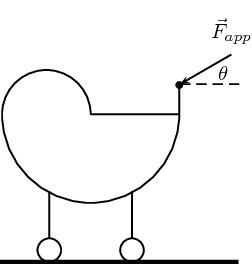\begin{align*} W&= F\Delta x \cos\theta \\ &= (50)(30)\cos(30)\\ & = \text{1 299,00}\text{ J} \end{align*} 1,30 kJ

How much work is done by the force required to raise a $$\text{2 000}$$ $$\text{N}$$ lift 5 floors vertically at a constant speed? The vertical distance between floors is $$\text{5}$$ $$\text{m}$$ high.

\begin{align*} W&= F\Delta x \cos\theta \\ &= (2000)(5\times 5) \cos(0) \\ &= 2000 \times 25 \\ &= \text{50 000}\text{ J} \end{align*} 50 000 J

A student with a mass of $$\text{60}$$ $$\text{kg}$$ runs up three flights of stairs in $$\text{15}$$ $$\text{s}$$, covering a vertical distance of $$\text{10}$$ $$\text{m}$$. Determine the amount of work done by the student to elevate her body to this height.

\begin{align*} W&= F\Delta x \cos\theta \\ &= mg \Delta x \cos \theta \\ &= (60)(9,8) (10) \\ &= \text{5 880}\text{ J} \end{align*} 5 880 J

### Net work (ESCMC)

We have only looked at a single force acting on an object. Sometimes more than one force acts at the same time (we dealt with this in Grade 11). We call the work done after taking all the forces into account the net work done. If there is only one force acting then the work it does, if any, is the net work done. In this case there are two equivalent approaches we can adopt to finding the net work done on the object. We can:

• Approach 1: calculate the work done by each force individually and then sum them taking the signs into account. If one force does positive work and another does the same amount of work but it is negative then they cancel out.
• Approach 2: calculate the resultant force from all the forces acting and calculate the work done using the resultant force. This will be equivalent to Approach 1. If the resultant force parallel to the direction of motion is zero, no net work will be done.

Remember that work done tells you about the energy transfer to or from an object by means of a force. That is why we can have zero net work done even if multiple large forces are acting on an object. Forces that result in positive work increase the energy of the object, forces that result in negative work reduce the energy of an object. If as much energy is transferred to an object as is transferred away then the final result is that the object gains no energy overall.

## Worked example 4: Approach 1, calculating the net work on a car

The same car is now accelerating forward, but friction is working against the motion of the car. A force of $$\text{300}$$ $$\text{N}$$ is applied forward on the car while it is travelling $$\text{20}$$ $$\text{m}$$ forward. A frictional force of $$\text{100}$$ $$\text{N}$$ acts to oppose the motion. Calculate the net work done on the car.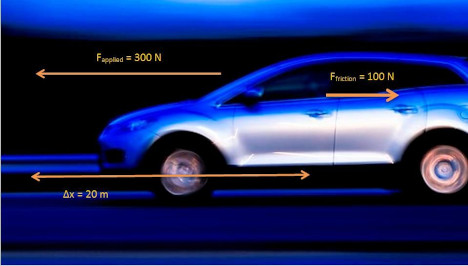Only forces with a component in the plane of motion are shown on the diagram. No work is done by $${F}_{g}$$ or $${F}_{\text{normal}}$$ as they act perpendicular to the direction of motion.

### Analyse the question to determine what information is provided

• The force applied is $$F_{\text{applied}}$$=$$\text{300}$$ $$\text{N}$$ forwards.

• The force of friction is $$F_{\text{friction}}$$=$$\text{100}$$ $$\text{N}$$ opposite to the direction of motion.

• The distance moved is $$\Delta x$$ = $$\text{20}$$ $$\text{m}$$.

• The applied force and distance moved are in the same plane so we can calculate the work done by the applied forward force and the work done by the force of friction backwards.

These quantities are all in the correct units, so no unit conversions are required.

### Analyse the question to determine what is being asked

• We are asked to find the net work done on the car. We know from the definition that work done is $$W={F}$$$$\Delta x$$ $$\cos\theta$$

### Next we calculate the work done by each force.

\begin{align*} W_{\text{applied}}& = {F}_{\text{applied}}\Delta x\cos\theta\\ & = \left(300\right)\left(20\right) \left(\cos 0\right)\\ & = \left(300\right)\left(20\right) \left(1\right)\\ & = \text{6 000}\text{ J} \end{align*}\begin{align*} W_{\text{friction}}& = {F}_{\text{friction}}\Delta x\cos\theta\\ & = \left(100\right)\left(20\right) \left(\cos 180\right)\\ & = \left(100\right)\left(20\right) \left(-1\right)\\ & = -\text{2 000}\text{ J} \end{align*}\begin{align*} {W}_{\text{net}}& = {W}_{\text{applied}} + {W}_{\text{friction}} \\ & = \left(6000\right) + \left(-2000\right) \\ & = \text{4 000}\text{ J} \end{align*}

The answer shown in this worked example shows that although energy has been lost by the car to friction, the total work done on the car has resulted in a net energy gain. This can be seen by the positive answer.

As mentioned before, there is an alternative method to solving the same problem, which is to determine the net force acting on the car and to use this to calculate the work. This means that the vector forces acting in the plane of motion must be added to get the net force $$\vec{F}_{\text{net}}$$. The net force is then applied over the displacement to get the net work $${W}_{\text{net}}$$.

## Worked example 5: Approach 2, calculating the net force

The same car is now accelerating forward, but friction is working against the motion of the car. A force of $$\text{300}$$ $$\text{N}$$ is applied forward on the car while it is travelling $$\text{20}$$ $$\text{m}$$ forward. A frictional force of $$\text{100}$$ $$\text{N}$$ acts to oppose the motion. Calculate the net work done on the car.

Only forces with a component in the plane of motion are shown on the diagram. No work is done by $${F}_{g}$$ or $${F}_{\text{normal}}$$ as they act perpendicular to the direction of motion. The net force acting in the plane of motion will be calculated using the non-perpendicular forces.

### Analyse the question to determine what information is provided

• The force applied is $$\vec{F}_{\text{applied}}$$=$$\text{300}$$ $$\text{N}$$ forwards.

• The force of friction is $$\vec{F}_{\text{friction}}$$=$$\text{100}$$ $$\text{N}$$ backwards.

• The distance moved is $$\Delta x$$ = $$\text{20}$$ $$\text{m}$$.

• The applied forces $$\vec{F}_{\text{applied}}= \text{300}\text{ N}$$ and the force of friction $$\vec{F}_{\text{friction}}= \text{100}\text{ N}$$ are in the same plane as the distance moved. Therefore, we can add the vectors. As vectors require direction, we will say that forward is positive and therefore backward is negative. Note, the force of friction is acting at $${180}^{0}$$ i.e. backwards and so is acting in the opposite vector direction i.e. negative.

These quantities are all in the correct units, so no unit conversions are required.

### Analyse the question to determine what is being asked

• We are asked to find the net work done on the car. We know from the definition that work done is $$W_{\text{net}}={F}_{\text{net}}$$$$\Delta x \cos \theta$$

### We calculate the net force acting on the car, and we convert this into net work.

First we draw the force diagram: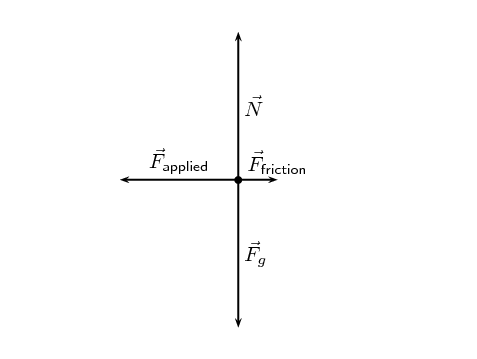Let forwards (to the left in the picture) be positive. We know that the motion of the car is in the horizontal direction so we can neglect the force due to gravity, $$\vec{F}_g$$, and the normal force, $$\vec{N}$$. Note: if the car were on a slope we would need to calculate the component of gravity parallel to the slope.

\begin{align*} \vec{F}_{\text{net}}& = \vec{F}_{\text{applied}} + \vec{F}_{\text{friction}}\\ & = \left(+300\right) + \left(-100\right)\\ \vec{F}_{\text{net}}& = \text{200}\text{ N}~\text{forwards} \end{align*}

$$\vec{F}_{\text{net}}$$ is pointing in the same direction as the displacement, therefore the angle between the force and displacement is $$\theta=0^\circ$$.

\begin{align*} {W}_{\text{net}}& = {F}_{\text{net}}\Delta x \cos \theta \\ & = \left(200\right)\left(20\right)\cos\left(0\right)\\ & = \text{4 000}\text{ J} \end{align*}

The two different approaches give the same result but it is very important to treat the signs correctly. The forces are vectors but work is a scalar so they shouldn't be interpreted in the same way.